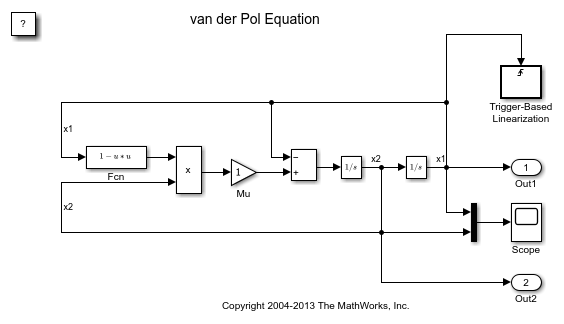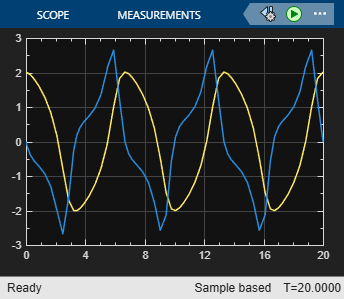# Generate Linear Models for a Rising Edge Trigger Signal

You can use state and simulation time logging to extract the model states at operating points. In this example, the model is configured to get the states when the `x1` signal triggers the Trigger-Based Linearization block on a rising edge.

In this model, the Trigger type of the Trigger-Based Linearization block is set to `rising`. On the Data Import/Export pane of the Model Configuration Parameters dialog box, the `States` and `Time` check boxes are selected.After simulating the model, the following variables appear in the MATLAB workspace:

• ex_vdp_triggered_linearization_Trigger_Based_Linearization

• tout

• xout

To get the index to the first operating point time, execute the following command:

```ind1 = find(ex_vdp_triggered_linearization_Trigger_Based_Linearization(1).OperPoint.t==tout); ```

To get the state vector at this operating point, execute the following command:

```x1 = xout(ind1,:); ```